# SAT Math Multiple Choice Question 11: Answer and Explanation

### Test Information

Question: 11

1. y = 3x - 1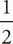y + x = 1

In the system of equations above, if (x, y) is the solution to the system, what is the value of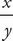?

• A.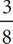• B.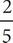• C.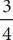• D.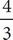C Start by multiplying the second equation by 2 to clear the fractions. The equation becomes y + 2x = 2. To get it into the same form as the other equations, subtract 2x from both sides to get y = -2x + 2. Set the two x expressions equal to get 3x - 1 = -2x + 2. Add 2x and 1 to both sides, so the equation becomes 5x = 3, then divide by 5 to find that x =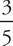. Plug this value into the y = 3x - 1 to get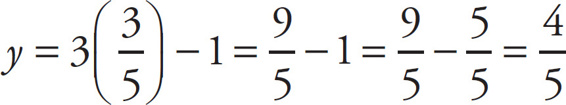. Finally, find the value of: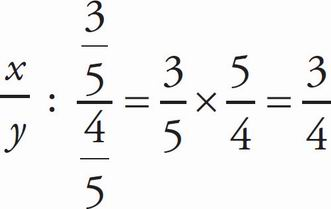, which is (C).